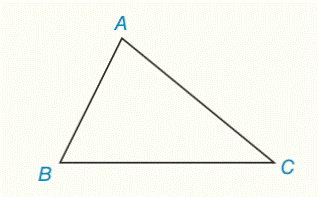Chapter 2.CR, Problem 41CRElementary Geometry For College St...

7th Edition
Alexander + 2 others
ISBN: 9781337614085

Solutions

Chapter
SectionElementary Geometry For College St...

7th Edition
Alexander + 2 others
ISBN: 9781337614085
Textbook Problem

Construct the line through C parallel to A B ¯ .To determine

To construct:

The line through C parallel to AB¯.

Explanation

Given:

A triangle ABC is given.

Figure (1)

Approach:

If two lines are cut by a transversal so that a pair of corresponding angles is congruent, then these lines are parallel.

Construction:

Step (1): Scribe an arbitrary arc on BC¯ from point B which intersect line BC¯ on point E and line AB¯ on point D.

Figure (2)

Step (2): Scribe an arc from point E which intersect AB¯ on point D

Still sussing out bartleby?

Check out a sample textbook solution.

See a sample solution

The Solution to Your Study Problems

Bartleby provides explanations to thousands of textbook problems written by our experts, many with advanced degrees!

Get Started

Subtract and check: 417,286287,156

Elementary Technical Mathematics

Evaluate the expression sin Exercises 116. (23)2

Finite Mathematics and Applied Calculus (MindTap Course List)

Define the terms population and sample, and explain the role of each in a research study.

Essentials of Statistics for The Behavioral Sciences (MindTap Course List)